In :
# %load ../../preconfig.py
%matplotlib inline
import matplotlib.pyplot as plt
import seaborn as sns
sns.set(color_codes=True)
plt.rcParams['axes.grid'] = False

#import numpy as np
#import pandas as pd

#import sklearn

#import itertools

import logging
logger = logging.getLogger()

def show_image(filename, figsize=None, res_dir=True):
if figsize:
plt.figure(figsize=figsize)

if res_dir:
filename = './res/{}'.format(filename)



# 11 Dimensionality Reduction¶

### 11.1 Eigenvalues and Eigenvectors of Symmetric Matrices¶

#### 11.1.1 Definitions¶

$M e = \lambda e$, where $\lambda$ is an eigenvalue and $e$ is the corresponding eigenvector.

to make the eigenvector unique, we require that:

1. every eigenvector is a unit vector.

2. the first nonzero component of an eigenvector is positive.

#### 11.1.2 Computing Eigenvalues and Eigenvectors¶

To find principal eigenvector, we use power iteration method:
start with any unit vector $v$, and compute $M^i v$ iteratively until it converges. When $M$ is a stochastic matrix, the limiting vector is the principal eigenvector, and its corresponding eigenvalue is 1.

Another method $O(n^3)$: algebra solution.

#### 11.1.3 Finding Eigenpairs by Power Iteration¶

idea:
Start by computing the pricipal eigenvector, then modify the matrix to, in effect, remove the principal eigenvector.

1. To find the pricipal eigenpair

power iteration:
Start with any nonzero vector $x_0$ and then iterate: $$x_{k+1} := \frac{M x_k}{\| M x_k\|}$$

$x$ is the pricipal eigenvector when it reaches convergence, and $\lambda_1 = x^T M x$.

Attention: Since power iteration will introduce small errors, inaccuracies accumulate when we try to compute all eigenpairs.

2. To find the second eigenpair

we create $M^* = M - \lambda_1 x x^T$, then use power iteration again.

proof: the second eigenpair of $M^*$ is also that of $M$.

#### 11.1.4 The Matrix of Eigenvectors¶

the eigenvectors of a symmetric matrix are orthonormal

### 11.2 Pricipal-Component Analysis¶

idea:
treat the set of tuples as a matrix $M$ and find the eigenvectors for $M M^T$ or $M^T M$.

In :
plt.imshow(plt.imread('./res/fig11_2.png'))

Out:
<matplotlib.image.AxesImage at 0x1173e1240>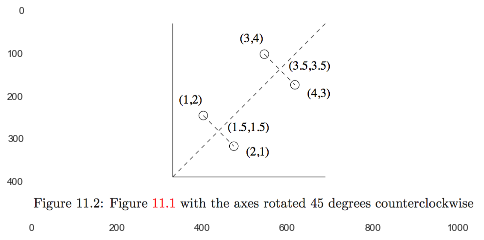#### 11.2.2 Using Eigenvectors for Dimensionality Reduction¶

Since $M^T M e = \lambda e = e \lambda$,
Let $E$ be the matrix whose columns are the eigenvectors, ordered as largest eigenvalue first. Define the matrix $L$ to have the eigenvalues of $M^T M$ along the diagonal, largest first, and 0's in all other entries.

$M^T M E = E L$

Let $E_k$ be the first $k$ columns of $E$. Then $M E_k$ is a $k$-dimensional representation of $M$.

#### 11.2.3 The Matrix of Distances¶

\begin{align} M^T M e &= \lambda e \\ M M^T (M e) &= M \lambda e = \lambda (M e) \end{align}

the eigenvalues of $M M^T$ are the eigenvalues of $M^T M$ plus additional 0's, and their eigenvectors are shared.

### 11.3 Singular-Value Decomposition¶

#### 11.3.1 Definition of SVD¶

Let $M$ be an $m \times n$ matrix, and let the rank of $M$ be $r$.

$$M = U \Sigma V^T$$
• $U$ is an $m \times r$ column-orthnormal matrix (each of its columns is a unit vector and the dot product of any two columns is 0).

• $V$ is an $n \times r$ column-orthnormal maxtrix.

• $\Sigma$ is a diagonal matrix. The elements of $\Sigma$ are called the singular values of $M$.

In :
show_image('fig11_5.png')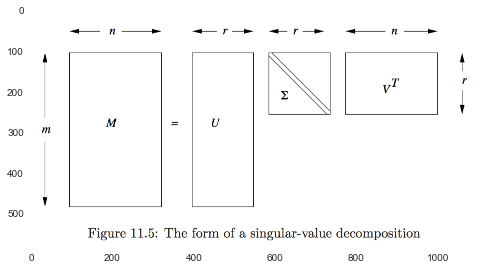In :
show_image('fig11_7.png', figsize=(8, 10))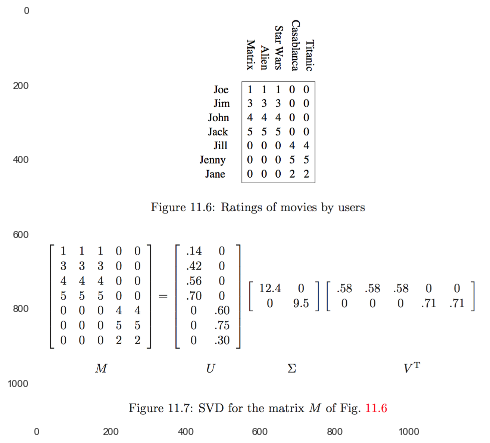#### 11.3.2 Interpretation of SVD¶

viewing the $r$ columns of $U$, $\Sigma$, and $V$ as representing concepts that are hidden in the original matrix $M$.

In Fig 11.7:

• concepts: "science fiction" and "romance".

• $U$ connects peopel to concepts.

• $V$ connects movies to concepts.

• $\Sigma$ give the strength of each of the concepts.

#### 11.3.3 Dimensionality Reduction Using SVD¶

In :
show_image('fig11_9.png', figsize=(8, 10))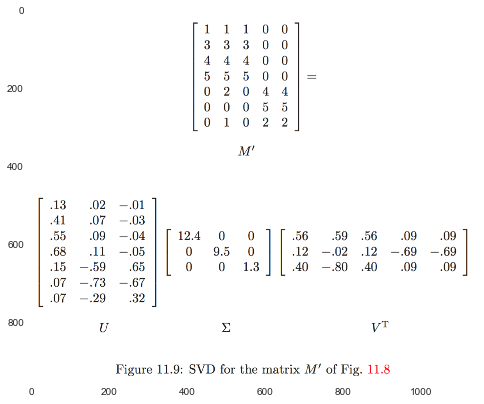If we set the $s$ smallest singular values to 0, then we can also eliminate the corresponding $s$ rows of $U$ and $V$.

In :
show_image('fig11_10.png', figsize=(8, 10))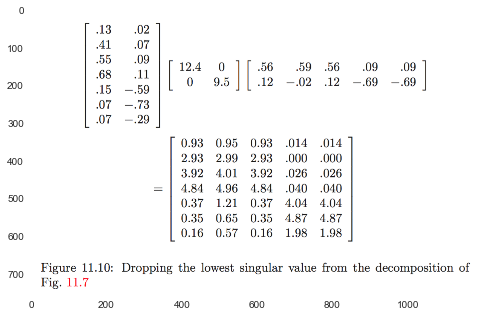#### 11.3.4 Why Zeroing Low Singular Values Works¶

Let $M = P Q R$, $m_{i j} = \sum_k \sum_l p_{ik} q_{kl} r_{lj}$.

Then \begin{align} \| M \|^2 &= \sum_i \sum_j (m_{ij})^2 \\ &= \sum_i \sum_j (\sum_k \sum_l p_{ik} q_{kl} r_{lj})^2 \\ &= \sum_i \sum_j \left ( \sum_k \sum_l \sum_n \sum_m p_{ik} q_{kl} r_{lj} p_{in} q_{nm} r_{mj} \right) \\ &\text{as $Q$ is diagonal matrix, $q_{kl}$ and $q_{nm}$ will be 0 unless $k = l$ and $n = m$.} \\ &= \sum_i \sum_j \sum_k \sum_n p_{ik} q_{kk} r_{kj} p_{in} q_{nn} r_{nj} \\ &= \sum_j \sum_k \sum_n \color{blue}{\sum_i p_{ik} p_{in}} q_{kk} r_{kj} q_{nn} r_{nj} \\ &\text{as } P = U, \sum_i p_{ik} p_{in} = 1 \text{ if } k = n \text{ and 0 otherwise} \\ &= \sum_j \sum_k q_{kk} r_{kj} q_{kk} r_{kj} \\ &= \sum_k (q_{kk})^2 \end{align}

How many singular values should we retain?

A useful rule of thumb is to retain enough singular values to make up 90\% of the energy in $\Sigma$.

#### 11.3.5 Querying Using Concepts¶

Let $q$ is the vector of user Quincy

• what movies he would like? "concept space": $q V$, select the one whose score is highest.

• find users similar to Quincy? measure the similarity of users by their cosine distance in concept space.

#### 11.3.6 Computing the SVD of a Matrix¶

The SVD of a matrix $M$ is strongly connected to the eigenvalues of the symmetric matrices $M^T M$and $M M^T$.

$M^T = (U \Sigma V^T)^T = V \Sigma^T U^T = V \Sigma U^T$

\begin{align} M^T M &= V \Sigma U^T U \Sigma V^T \\ &= V \Sigma^2 V^T \\ M^T M V &= V \Sigma^2 V^T V \\ & = V \Sigma^2 \end{align}

similar, $M M^T U = U \Sigma^2$.

### 11.4 CUR Decomposition¶

SVD: even if $M$ is sparse, $U$ and $V$ will be dense.

CUR: if $M$ is sparse, $C$ and $R$ will be sparse.

#### 11.4.2 Choosing Rows and Columns Properly¶

Let $f = \sum_{i,j} m_{ij}^2$.

1. find $C$

• pick $r$ rows, and each row is picked with $p_i = \frac{\sum_j m_{ij}^2}{f}$.
• normailize: each row is divided by $\sqrt{r p_i}$.
2. find $R$ selected in the analogous way.

3. Counstructing $U$.

• find $M$, that is the intersection of the chosen columns of $C$ and $R$.
• compute the SVD of $W$: $W = X \Sigma Y^T$.
• compute $\Sigma^+$, the Moore-Penrose pseudoinverse of the diagonal matrix $\Sigma$: replace $\sigma$ by $1/\sigma$ if $\sigma \neq 0$.
• $U = Y (\Sigma^+)^2 X^T$.
In :
show_image('fig11_13.png')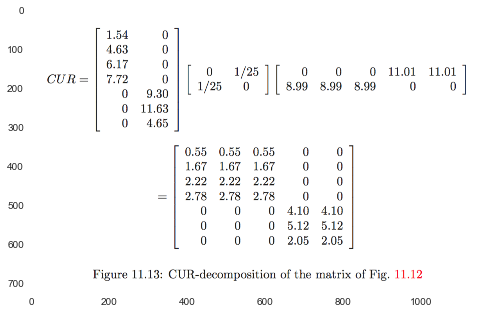##### Eliminating Duplicate Rows and Columns¶

it is possible that a single row or column is selected more than once, how to deal with it?

• let it go.

• or, merge same rows and/or columns $W$ will not be a sqaure matrix, then we need transpose the result to get $\Sigma^+$.

In :
show_image('ex11_17.png')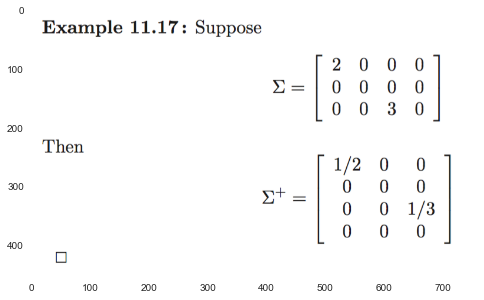In :
#Exercise

In [ ]: